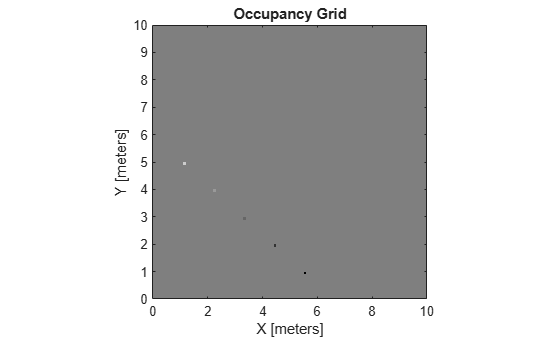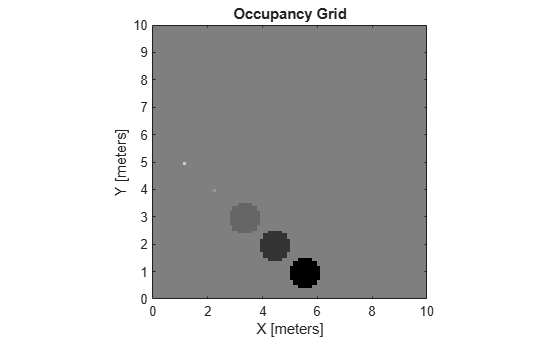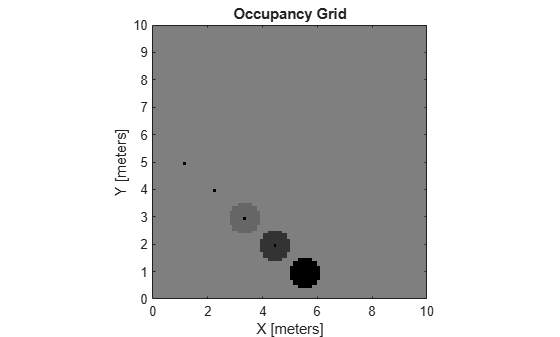Documentation

# setOccupancy

Set occupancy value of locations

## Syntax

``setOccupancy(map,xy,occval)``
``setOccupancy(map,xy,occval,"local")``
``setOccupancy(map,ij,occval,"grid")``
``validPts = setOccupancy(___)``
``setOccupancy(map,bottomLeft,inputMatrix)``
``setOccupancy(map,bottomLeft,inputMatrix,"local")``
``setOccupancy(map,topLeft,inputMatrix,"grid")``

## Description

````setOccupancy(map,xy,occval)` assigns the occupancy values to each coordinate specified in `xy`. `occval` can be a column vector the same size of `xy` or a scalar, which is applied to all coordinates.```
````setOccupancy(map,xy,occval,"local")` assigns occupancy values, `occval`, to the input array of local coordinates, `xy`, as local coordinates.```

example

````setOccupancy(map,ij,occval,"grid")` assigns occupancy values, `occval`, to the input array of grid indices, `ij`, as `[rows cols]`.```
````validPts = setOccupancy(___)` outputs an `n`-element vector of logical values indicating whether input coordinates are within the map limits.```
````setOccupancy(map,bottomLeft,inputMatrix)` assigns a matrix of occupancy values by specifying the bottom-left corner location in world coordinates.```
````setOccupancy(map,bottomLeft,inputMatrix,"local")` assigns a matrix of occupancy values by specifying the bottom-left corner location in local coordinates.```
````setOccupancy(map,topLeft,inputMatrix,"grid")` assigns a matrix of occupancy values by specifying the top-left cell index in grid indices and the matrix size.```

## Examples

collapse all

Create a 10 m-by-10 m empty map.

`map = occupancyMap(10,10,10);`

Update the occupancy of world locations with specific probability values and display the map.

```x = [1.2; 2.3; 3.4; 4.5; 5.6]; y = [5.0; 4.0; 3.0; 2.0; 1.0]; pvalues = [0.2 0.4 0.6 0.8 1]; updateOccupancy(map,[x y],pvalues) figure show(map)```Inflate occupied areas by a radius of 0.5 m. Larger occupancy values overwrite the smaller values.

```inflate(map,0.5) figure show(map)```Get grid locations from world locations.

`ij = world2grid(map,[x y]);`

Set grid locations to occupied locations.

```setOccupancy(map,ij,ones(5,1),'grid') figure show(map)```## Input Arguments

collapse all

Map representation, specified as a `occupancyMap` object. This object represents the environment of the vehicle. The object contains a matrix grid with values representing the probability of the occupancy of that cell. Values close to 1 represent a high probability that the cell contains an obstacle. Values close to 0 represent a high probability that the cell is not occupied and obstacle free.

World coordinates, specified as an n-by-2 matrix of ```[x y]``` pairs, where n is the number of world coordinates.

Data Types: `double`

Grid positions, specified as an n-by-2 matrix of ```[i j]``` pairs in ```[rows cols]``` format, where n is the number of grid positions.

Data Types: `double`

Probability occupancy values, specified as a scalar or a column vector the same size as either `xy` or `ij`. A scalar input is applied to all coordinates in either `xy` or `ij`.

Values close to 0 represent a high probability that the cell is not occupied and obstacle free.

Occupancy values, specified as a matrix. Values are given between 0 and 1 inclusively.

Location of bottom left corner of output matrix in world or local coordinates, specified as a two-element vector, ```[xCoord yCoord]```. Location is in world or local coordinates based on syntax.

Data Types: `double`

Location of top left corner of grid, specified as a two-element vector, `[iCoord jCoord]`.

Data Types: `double`

## Output Arguments

collapse all

Valid map locations, returned as an n-by-1 column vector equal in length to `xy` or `ij`. Locations inside the map return a value of 1. Locations outside the map limits return a value of 0.

## Limitations

Occupancy values have a limited resolution of ±0.001. The values are stored as `int16` using a log-odds representation. This data type limits resolution, but saves memory when storing large maps in MATLAB®. When calling `setOccupancy` and then `getOccupancy`, the value returned might not equal the value you set. For more information, see the log-odds representations section in Occupancy Grids.